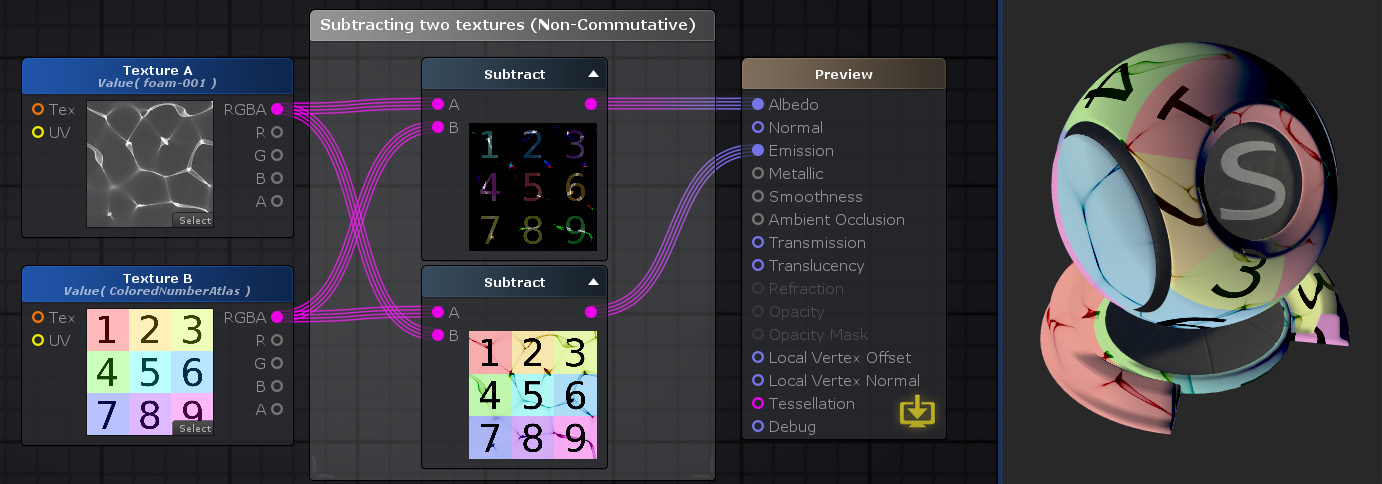## Subtract Node

The Subtract Node ( shortcut: S key ) does an arithmetic subtraction where A is subtracted by B or ( A - B ).

Subtraction between multi-channel data types are done per-channel. If A and B have different channel amounts a cast is done to match the one with the most amount of channels.

Note: Subtraction is not commutative so value's order is important.Nodes used: Texture Sample, Subtract

Node Parameter Description Default Value
A The minuend, the value you want to subtract from. Only visible if the respective input port is not connected. 0
B The subtrahend, the value you want to be subtracted by. Only visible if the respective input port is not connected. 0

Input Port Description Type
A The minuend, the value you want to subtract from. Float 
B The subtrahend, the value you want to be subtracted by. Float 

1. ^ Port automatically adapts to all connection types except Matrices and Sampler types.

 Math Operators - Subtract/Divide